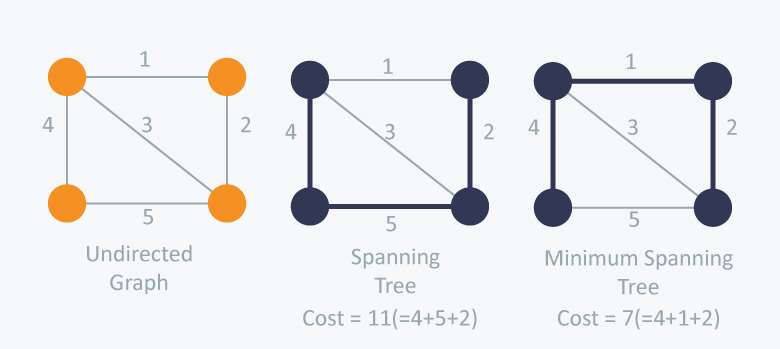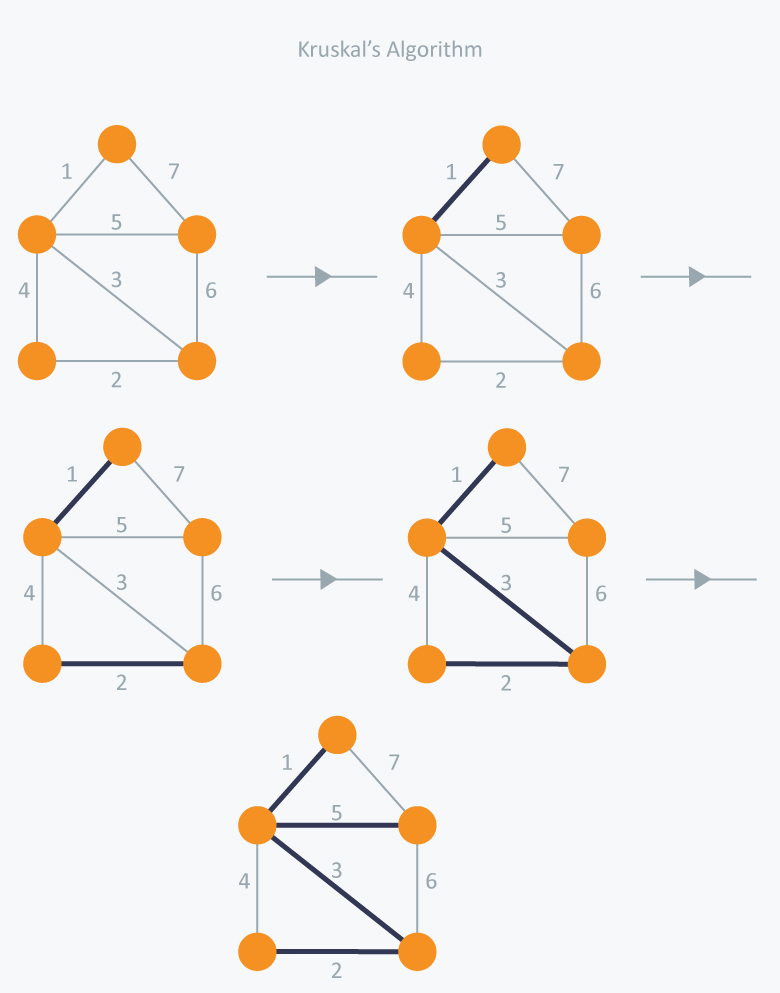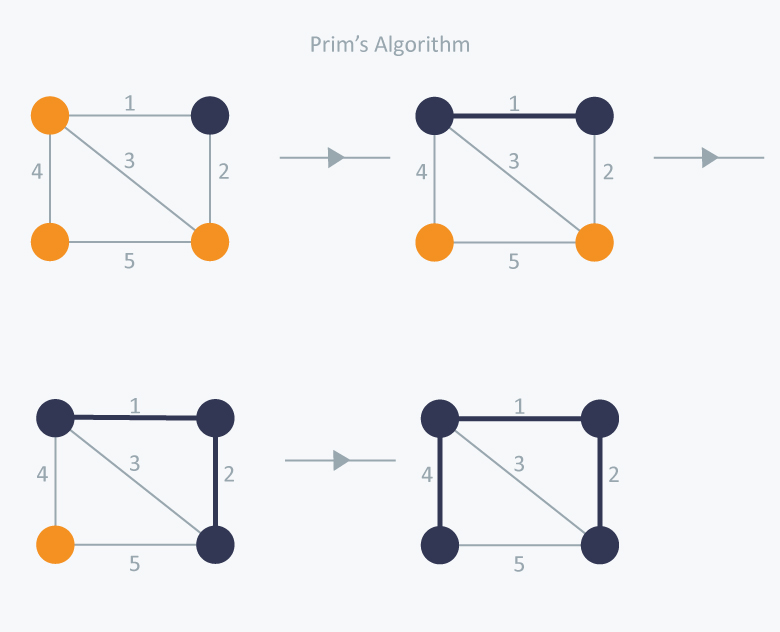Algorithms
Topics:
Minimum Spanning Tree
• Searching
• Sorting
• Greedy Algorithms
• Graphs
• String Algorithms
• Dynamic Programming

# Minimum Spanning Tree

Pre-requisites: Graphs, Trees

# What is a Spanning Tree?

Given an undirected and connected graph $G = (V, E)$, a spanning tree of the graph $G$ is a tree that spans $G$ (that is, it includes every vertex of $G$) and is a subgraph of $G$ (every edge in the tree belongs to $G$)

# What is a Minimum Spanning Tree?

The cost of the spanning tree is the sum of the weights of all the edges in the tree. There can be many spanning trees. Minimum spanning tree is the spanning tree where the cost is minimum among all the spanning trees. There also can be many minimum spanning trees.

Minimum spanning tree has direct application in the design of networks. It is used in algorithms approximating the travelling salesman problem, multi-terminal minimum cut problem and minimum-cost weighted perfect matching. Other practical applications are:

1. Cluster Analysis
2. Handwriting recognition
3. Image segmentationThere are two famous algorithms for finding the Minimum Spanning Tree:

# Kruskal’s Algorithm

Kruskal’s Algorithm builds the spanning tree by adding edges one by one into a growing spanning tree. Kruskal's algorithm follows greedy approach as in each iteration it finds an edge which has least weight and add it to the growing spanning tree.

Algorithm Steps:

• Sort the graph edges with respect to their weights.
• Start adding edges to the MST from the edge with the smallest weight until the edge of the largest weight.
• Only add edges which doesn't form a cycle , edges which connect only disconnected components.

So now the question is how to check if $2$ vertices are connected or not ?

This could be done using DFS which starts from the first vertex, then check if the second vertex is visited or not. But DFS will make time complexity large as it has an order of $O(V + E)$ where $V$ is the number of vertices, $E$ is the number of edges. So the best solution is "Disjoint Sets":
Disjoint sets are sets whose intersection is the empty set so it means that they don't have any element in common.

Consider following example:In Kruskal’s algorithm, at each iteration we will select the edge with the lowest weight. So, we will start with the lowest weighted edge first i.e., the edges with weight 1. After that we will select the second lowest weighted edge i.e., edge with weight 2. Notice these two edges are totally disjoint. Now, the next edge will be the third lowest weighted edge i.e., edge with weight 3, which connects the two disjoint pieces of the graph. Now, we are not allowed to pick the edge with weight 4, that will create a cycle and we can’t have any cycles. So we will select the fifth lowest weighted edge i.e., edge with weight 5. Now the other two edges will create cycles so we will ignore them. In the end, we end up with a minimum spanning tree with total cost 11 ( = 1 + 2 + 3 + 5).

Implementation:

#include <iostream>
#include <vector>
#include <utility>
#include <algorithm>

using namespace std;
const int MAX = 1e4 + 5;
int id[MAX], nodes, edges;
pair <long long, pair<int, int> > p[MAX];

void initialize()
{
for(int i = 0;i < MAX;++i)
id[i] = i;
}

int root(int x)
{
while(id[x] != x)
{
id[x] = id[id[x]];
x = id[x];
}
return x;
}

void union1(int x, int y)
{
int p = root(x);
int q = root(y);
id[p] = id[q];
}

long long kruskal(pair<long long, pair<int, int> > p[])
{
int x, y;
long long cost, minimumCost = 0;
for(int i = 0;i < edges;++i)
{
// Selecting edges one by one in increasing order from the beginning
x = p[i].second.first;
y = p[i].second.second;
cost = p[i].first;
// Check if the selected edge is creating a cycle or not
if(root(x) != root(y))
{
minimumCost += cost;
union1(x, y);
}
}
return minimumCost;
}

int main()
{
int x, y;
long long weight, cost, minimumCost;
initialize();
cin >> nodes >> edges;
for(int i = 0;i < edges;++i)
{
cin >> x >> y >> weight;
p[i] = make_pair(weight, make_pair(x, y));
}
// Sort the edges in the ascending order
sort(p, p + edges);
minimumCost = kruskal(p);
cout << minimumCost << endl;
return 0;
}


Time Complexity:
In Kruskal’s algorithm, most time consuming operation is sorting because the total complexity of the Disjoint-Set operations will be $O(E log V)$, which is the overall Time Complexity of the algorithm.

# Prim’s Algorithm

Prim’s Algorithm also use Greedy approach to find the minimum spanning tree. In Prim’s Algorithm we grow the spanning tree from a starting position. Unlike an edge in Kruskal's, we add vertex to the growing spanning tree in Prim's.

Algorithm Steps:

• Maintain two disjoint sets of vertices. One containing vertices that are in the growing spanning tree and other that are not in the growing spanning tree.
• Select the cheapest vertex that is connected to the growing spanning tree and is not in the growing spanning tree and add it into the growing spanning tree. This can be done using Priority Queues. Insert the vertices, that are connected to growing spanning tree, into the Priority Queue.
• Check for cycles. To do that, mark the nodes which have been already selected and insert only those nodes in the Priority Queue that are not marked.

Consider the example below:In Prim’s Algorithm, we will start with an arbitrary node (it doesn’t matter which one) and mark it. In each iteration we will mark a new vertex that is adjacent to the one that we have already marked. As a greedy algorithm, Prim’s algorithm will select the cheapest edge and mark the vertex. So we will simply choose the edge with weight 1. In the next iteration we have three options, edges with weight 2, 3 and 4. So, we will select the edge with weight 2 and mark the vertex. Now again we have three options, edges with weight 3, 4 and 5. But we can’t choose edge with weight 3 as it is creating a cycle. So we will select the edge with weight 4 and we end up with the minimum spanning tree of total cost 7 ( = 1 + 2 +4).

Implementation:

#include <iostream>
#include <vector>
#include <queue>
#include <functional>
#include <utility>

using namespace std;
const int MAX = 1e4 + 5;
typedef pair<long long, int> PII;
bool marked[MAX];

long long prim(int x)
{
priority_queue<PII, vector<PII>, greater<PII> > Q;
int y;
long long minimumCost = 0;
PII p;
Q.push(make_pair(0, x));
while(!Q.empty())
{
// Select the edge with minimum weight
p = Q.top();
Q.pop();
x = p.second;
// Checking for cycle
if(marked[x] == true)
continue;
minimumCost += p.first;
marked[x] = true;
for(int i = 0;i < adj[x].size();++i)
{
if(marked[y] == false)
}
}
return minimumCost;
}

int main()
{
int nodes, edges, x, y;
long long weight, minimumCost;
cin >> nodes >> edges;
for(int i = 0;i < edges;++i)
{
cin >> x >> y >> weight;
}
// Selecting 1 as the starting node
minimumCost = prim(1);
cout << minimumCost << endl;
return 0;
}


Time Complexity:
The time complexity of the Prim’s Algorithm is $O((V + E)logV)$ because each edge is inserted in the priority queue only once and insertion in priority queue take logarithmic time.

Contributed by: omar khaled abdelaziz abdelnabi